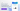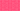Pricing# How to Calculate ARR (Accounting Rate of Return)?

Written by

If you’re making a long-term investment in an asset or project, it’s important to keep a close eye on your plans and budgets. Accounting Rate of Return (ARR) is one of the best ways to calculate the potential profitability of an investment, making it an effective means of determining which capital asset or long-term project to invest in. But how do you do an ARR calculation? Find out everything you need to know about the Accounting Rate of Return formula and how to calculate ARR, right here.

## What is ARR?

Accounting Rate of Return (ARR) is the percentage rate of return that is expected from an investment or asset compared to the initial cost of investment. Typically, ARR is used to make capital budgeting decisions. For example, if your business needs to decide whether to continue with a particular investment, whether it’s a project or an acquisition, an ARR calculation can help to determine whether going ahead is the right move.

## What is the Accounting Rate of Return formula?

The Accounting Rate of Return formula is as follows:

• ARR = average annual profit / average investment

Of course, that doesn’t mean too much on its own, so here’s how to put that into practice and actually work out the profitability of your investments.

## How to calculate ARR

Doing an ARR calculation is relatively simple. Here’s what you need to do to calculate ARR:

1. First off, work out the annual net profit of your investment. This will be the revenue remaining after all operating expenses, taxes, and interest associated with implementing the investment or project have been deducted.

2. If the investment is a fixed asset, such as property, you’ll need to work out the depreciation expense.

3. Then, to arrive at the final figure for annual net profit, simply subtract the depreciation expense from your annual revenue figure.

4. Finally, you simply divide the annual net profit by the initial cost of the asset or investment. The calculation will show a decimal, so multiply the result by 100 to see the percentage return.

If you’re not comfortable working this out for yourself, you can use an ARR calculator online to be extra sure that your figures are correct. EasyCalculation offers a simple tool for working out your ARR, although there are many different ARR calculators online to explore.

## ARR Calculation – an example

Here’s an example of how to use the Accounting Rate of Return formula in the real world. A Company wants to invest in new set of vehicles for the business. The vehicles cost £350,000 and would increase the company’s annual revenue by £100,000, as well as the company’s annual expenses by £10,000. The vehicles are estimated to have a useful shelf life of 20 years, with no salvage value. So, the ARR calculation is as follows:

1. Average annual profit = £100,000 - £10,000 = £90,000

2. Depreciation expense = £350,000 / 20 = £17,500

3. True average annual profit = £90,000 - £17,500 = £72,500

4. ARR = £72,500 / 350,000 = 0.2071 = 20.71%

So, in this example, for every pound that your company invests, it will receive a return of 20.71p. That’s relatively good, and if it’s better than the company’s other options, it may convince them to go ahead with the investment.

## How to calculate Accounting Rate of Return in Excel?

To keep track of ARR more comprehensively, it may be a good idea to use a spreadsheet tool like Microsoft Excel. If you’re using Excel to calculate ARR, follow these simple steps:

1. In A1, write ‘Year’.

2. In C1-G1, write 1, 2, 3, 4, 5 (assuming a five-year project).

3. In A2, write ‘Net Income’.

4. In C2-G2, write the net annual income for each year.

5. In A3, write ‘Initial Investment’.

6. In B3, write the initial investment for the project.

7. In A4, write ‘Salvage Value’.

8. In B4, write the salvage value, if any.

9. In A5, write ‘ARR’.

10. In B5, write =AVERAGE(C2:G2)/AVERAGE(B3:B4).

11. Press enter to calculate ARR.

12. ARR will now show in B5.

Remember that you may need to change these details depending on the specifics of your project. For example, your project may last longer than five years. Overall, however, this is a simple and efficient method for anyone who wants to learn how to calculate Accounting Rate of Return in Excel.

## We can help

If you’re making long-term investments, it’s important that you have a healthy cash flow to deal with any unforeseen events. Find out how GoCardless can help you with ad hoc payments or recurring payments.